# Forces on a slider crank

icecats
I am in a mechanical design class that has been focusing on the slider crank mechanism. My professor tends to just provide derived equations without showing the analysis. I feel like I am missing out on some key understanding because of this. Specifically, I am trying to do what should be a simple force analysis on a simplified slider crank. However, my results are not matching up with what the professors equations suggest.
In the attached pictures, Case 1 shows the slider crank in one position and Case 2 shows it in a different position. Based on a statics force analysis, I would expect that, for the same torque T, the equilibrium force F in Case 1 would be higher than Case 2. In fact, I would expect that F in Case 2 would be 0. However, the equations provided in class and intuition from the operation of a slider crank suggest the opposite. What am I doing wrong? I feel like it has something to do with the fact that the joints are pin joints and not rigid.
Thanks!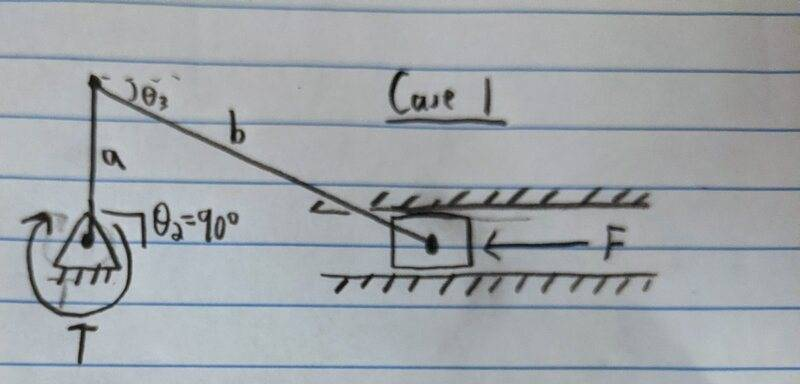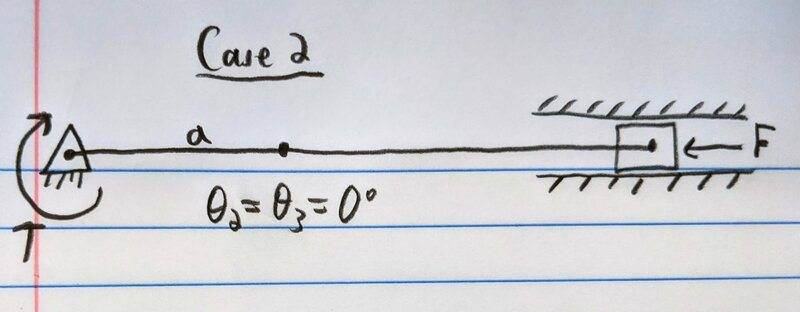Homework Helper
Gold Member
... Based on a statics force analysis, I would expect that, for the same torque T, the equilibrium force F in Case 1 would be higher than Case 2.
...
Could you show us that statics force analysis?

The magnitude of the tangential force perpendicular to a is constant during a full rotation.
As its direction constantly changes respect to the axis of freedom of the piston, it is a pure geometrical problem of vectors.

You can also analyze it as work in and work out of the mechanism.
The input work done by the shaft is constant; therefore, the output work at the piston (force x slid distance) should be equal, assuming no mechanical loses.

When reaching zero degrees or TDC, the traveled distance by the piston tends to zero; hence, the force should tend to...
When reaching 90 degrees, the slid distance by the piston is the greatest of that stroke; hence, the force should tend to...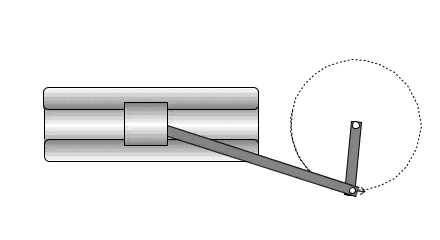Last edited:
icecats
Could you show us that statics force analysis?

The magnitude of the tangential force perpendicular to a is constant during a full rotation.
As its direction constantly changes respect to the axis of freedom of the piston, it is a pure geometrical problem of vectors.

You can also analyze it as work in and work out of the mechanism.
The input work done by the shaft is constant; therefore, the output work at the piston (force x slid distance) should be equal, assuming no mechanical loses.

When reaching zero degrees or TDC, the traveled distance by the piston tends to zero; hence, the force should tend to...
When reaching 90 degrees, the slid distance by the piston is the greatest of that stroke; hence, the force should tend to...

View attachment 292285
I have attached the statics force analysis. For Case 1, I would expect the force F to be a maximum. For Case 2, I would expect the force F to be 0 because there is no component of applied force in the x-direction.

Following the work relation, I would expect:
• When reaching zero degrees or TDC, the traveled distance by the piston tends to zero; hence, the force should tend to... be the highest.
• When reaching 90 degrees, the slid distance by the piston is the greatest of that stroke; hence, the force should tend to... be the lowest (tend towards 0).
This seemingly contradicts my statics analysis.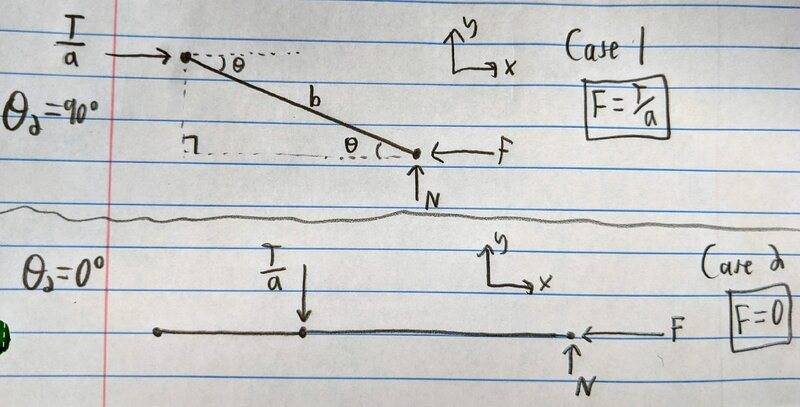Homework Helper
Gold Member
Last edited:
•icecats
Homework Helper
Gold Member
Based on a statics force analysis, I would expect that, for the same torque T, the equilibrium force F in Case 1 would be higher than Case 2. In fact, I would expect that F in Case 2 would be 0.
That is the case when the system is in static equilibrium, however I would imagine that the professor's equations are for the case when the crank is rotating.

So you need to throw away your intuition regarding the static case and replace it with intuition about the case when the crank is rotating and the slider is moving backwards and forwards. Can you describe that motion?

icecats
Exactly!
Only that at 90 degrees the force does not need to be zero, only at its minimum value.
Back to the drafting board, but this time using 10 degrees rather than zero.
Hint:
https://www.engineeringtoolbox.com/toggle-joint-d_2077.html
The toggle joint makes intuitive sense to me. I was able to work out the static analysis and derive the same equation. Thanks for your help!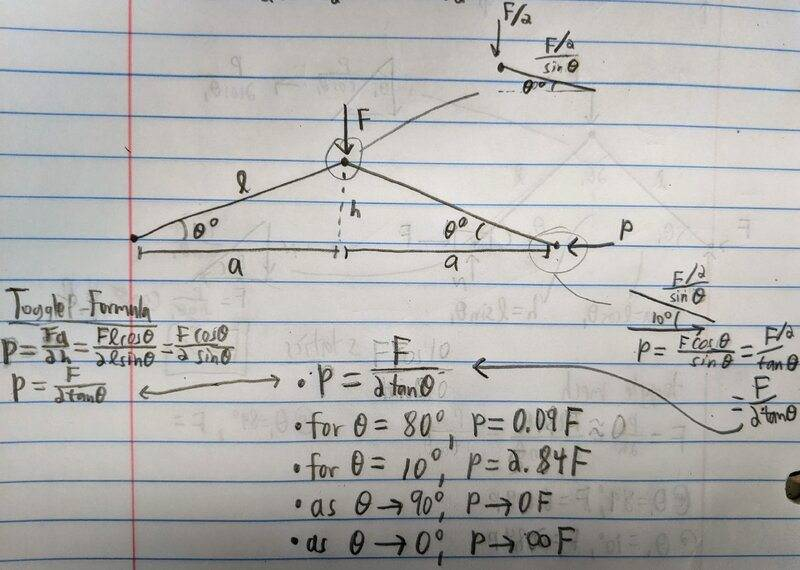That is the case when the system is in static equilibrium, however I would imagine that the professor's equations are for the case when the crank is rotating.

So you need to throw away your intuition regarding the static case and replace it with intuition about the case when the crank is rotating and the slider is moving backwards and forwards. Can you describe that motion?
I was assuming the case where the mechanism is in static equilibrium; that is, the force against the block is enough to balance the input torque.

Homework Helper
Gold Member
The instantaneous displacement/velocity of the sliding piston is always equal to the component in that same direction of the instantaneous tangential displacement/velocity of the ab joint.
Regarding instantaneous torques and forces, you must consider the mechanical advantage that the instantaneous geometry of the mechanism allows.

For more complicated mechanisms, your teacher may teach you the following method soon:
http://www.u.arizona.edu/~pen/ame352/Notes PDF/5.3 Velocity-graphical.pdf

http://alistairstutorials.co.uk/tutorial14.html